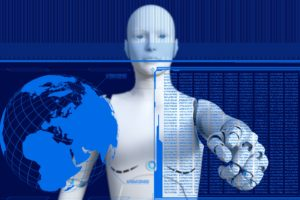## Purpose of Functions

### Learning Objectives

• Explain how equations and functions are used to describe relationships (such as cause and effect)Figure 1. Math and Predictions. We can make predictions in economics by applying mathematical concepts.

Often, economic models are expressed in terms of mathematical functions. What’s a function? Basically, a function describes a relationship involving one or more variables. Sometimes the relationship is a definition. For example (using words), Joan of Arc is a professor. This could be expressed as Joan of Arc = professor. Or, food = cherries, cheese, and chocolate means that cherries, cheese, and chocolate are food.

In economics, functions frequently describe cause and effect. The variable on the left-hand side is what is being explained (“the effect”). On the right-hand side is what’s doing the explaining (“the causes”). Functions are also useful for making predictions. For example, think about your grade in this course. We might be able to predict how well you will do in this course by considering how well you’ve done in other courses, by how much you attend class or participate in the online activities, and by how many hours you study.

Not all of those things will have equal impact on your grade. Let’s assume that your study time is most important and will have twice as much impact as the other factors. We are trying to describe 100 percent of the impact, so study time will explain 50 percent, attendance and participation will explain 25 percent, and your prior class grades will describe 25 percent. Together, this adds up to 100 percent.

Now, let’s turn that into a function. Your grade in the course can be represented as the following:

Grade = (0.50 x hours spent studying) + (0.25 x class attendance hours) + (0.25 x prior GPA)

### Try It

Economic models tend to express relationships using economic variables, such as:

Budget = money spent on econ books + money spent on music

(assuming that the only things you buy are economics books and music). Often, there is some assumption that has to be explained in order to identify where the model has been simplified.

As you can see, in economic models the math isn’t difficult. It’s used to help describe and explain the relationships between variables.

### Glossary

function:
a relationship or expression involving one or more variables

## Contribute!

Did you have an idea for improving this content? We’d love your input.